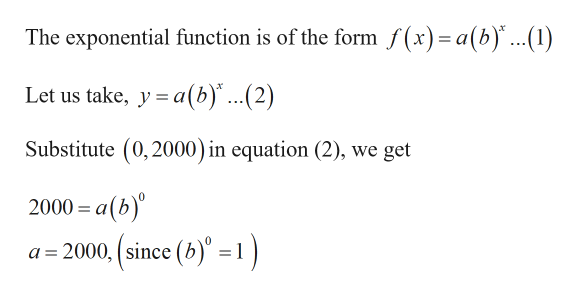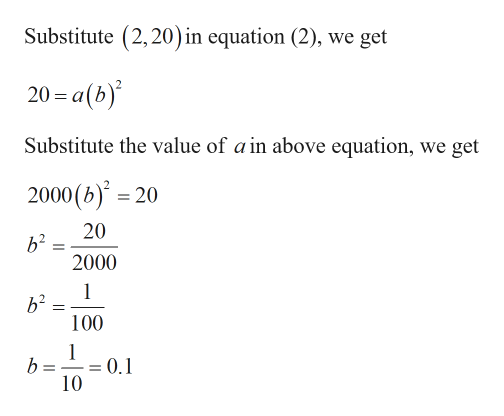# Find the equation of the exponential function that goes through the points (0,2000) and (2,20).

Question
10 views

Find the equation of the exponential function that goes through the points (0,2000) and (2,20).

check_circle

Step 1

Given:

Step 2

Calculation:help_outlineImage TranscriptioncloseThe exponential function is of the form f(x)=a(b)..(1) Let us take, y a(b)" ...(2) Substitute (0,2000) in equation (2), we get 2000= a(b) a 2000, (since (b)" =1) fullscreen
Step 3help_outlineImage TranscriptioncloseSubstitute (2,20) in equation (2), we get 20-a(b) Substitute the value of a in above equation, we get 2000 (b)20 20 b2 2000 1 b2 100 1 b = = 0.1 10 fullscreen

### Want to see the full answer?

See Solution

#### Want to see this answer and more?

Solutions are written by subject experts who are available 24/7. Questions are typically answered within 1 hour.*

See Solution
*Response times may vary by subject and question.
Tagged in

### Algebra